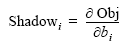Returns the shadow prices, or dual values, for the constraints at the optimal solution.

The shadow price is the amount by which the objective function changes when the constraint is altered by increasing its right-hand side coefficient, bi, by one unit. It is valid only for small changes in bi, and mathematically is defined as:This is the partial derivative of the objective function relative to the constraint RHS coefficient.

For a '<=' constraint and maximization problem, a shadow price indicates the amount the objective function improves when the constraint is relaxed. Shadow prices are usually meaningful when you are thinking of them in these terms. However, you should pay attention to the partial derivative definition to get the sign right.

The shadow can only be computed for continuous optimization problems. The shadow price does not exist for integer or mixed-integer optimizations, so can only be computed if every variable in the optimization problem is continuous.

For continuous problems, whether the shadow price can be computed depends upon the problem type and solver engine used. The following table summarizes the combinations for which shadow price can be computed (QP = quadratic objective + linear constraints, QCP = quadratically constrained):

"Problem Type"
Engine LP QP QCP NLP
SOCP Barrier Y Y N -
GRG Nonlinear Y Y Y Y
Evolutionary N N N N
LSLP Y - - -
LSGRG Y Y Y Y
LSSQP Y Y Y -
OptQuest N N N N
MOSEK Y Y N N
Knitro Y Y Y Y
XPress Y - - -
Gurobi Y - - -

This table may not be 100% accurate. [To do: empirically validate these entries]